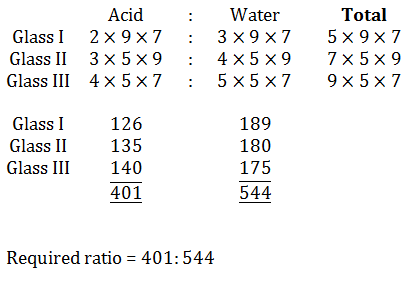Courses

# NABARD Quantitative Aptitude Practice: 4

## 30 Questions MCQ Test NABARD Assistant Manager Grade A Mock Test Series | NABARD Quantitative Aptitude Practice: 4

Description
This mock test of NABARD Quantitative Aptitude Practice: 4 for Banking Exams helps you for every Banking Exams entrance exam. This contains 30 Multiple Choice Questions for Banking Exams NABARD Quantitative Aptitude Practice: 4 (mcq) to study with solutions a complete question bank. The solved questions answers in this NABARD Quantitative Aptitude Practice: 4 quiz give you a good mix of easy questions and tough questions. Banking Exams students definitely take this NABARD Quantitative Aptitude Practice: 4 exercise for a better result in the exam. You can find other NABARD Quantitative Aptitude Practice: 4 extra questions, long questions & short questions for Banking Exams on EduRev as well by searching above.
QUESTION: 1

### Study the following table carefully to answer these questions: Table giving the number of candidates appeared in the examination and percentage of students passed from various institutes over the years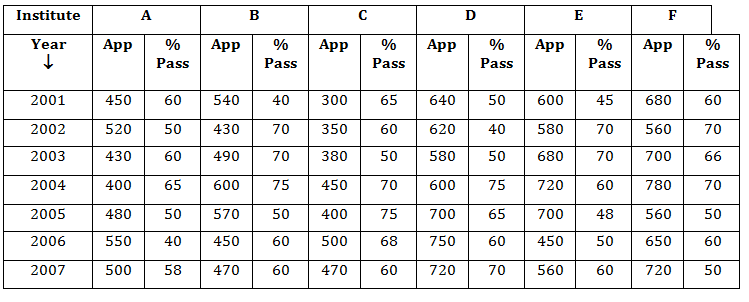Q. What is the total number of students passed from all institutes together in the year 2006?

Solution:

Total number of students passed from all institutes in the year 2006
= 40% of 550 + 60% of 450 + 68% of 500 + 60% of 750 + 50% of 450 + 60% of 650
= 220 + 270 + 340 + 450 + 225 + 390
= 1895

QUESTION: 2

### Study the following table carefully to answer these questions: Table giving the number of candidates appeared in the examination and percentage of students passed from various institutes over the yearsQ. Approximately, what is the overall percentage of students passed from institute C for all the years?

Solution:

Total students passed from C from 2001 to 2007
= 65% of 300 + 60% of 350 + 50% of 380
+ 70% of 450 + 75% of 400 + 68% of 500 + 60% of 470
= 195 + 210 + 190 + 315 + 300 + 340 + 282
= 1832
Total student appeared from C = 300 + 350 + 380 + 450 + 400 + 500 + 470
= 2850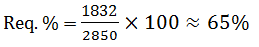QUESTION: 3

### Study the following table carefully to answer these questions: Table giving the number of candidates appeared in the examination and percentage of students passed from various institutes over the yearsQ. What is the ratio of the number of students passed from institute F in 2003 to the number of students passed from institute B in 2005?

Solution: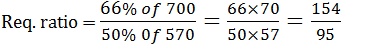QUESTION: 4

Study the following table carefully to answer these questions:

Table giving the number of candidates appeared in the examination and percentage of students passed from various institutes over the yearsQ. What is the ratio of the average number of students appeared from institute A for all the years to that from institute D?

Solution: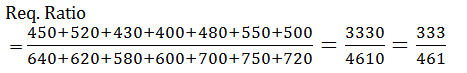QUESTION: 5

Study the following table carefully to answer these questions:

Table giving the number of candidates appeared in the examination and percentage of students passed from various institutes over the yearsQ. What is the overall percentage of students passed from all the institutes together in 2004? (rounded off to the nearest integer)

Solution:

Total number of students passed from all institute in 2004.
= 65% of 400 + 75% of 600 + 70% of 450 + 75% of 600 + 60% of 720 + 70% of 780
= 260 + 450 + 315 + 450 + 432 + 546 = 2453
Total students appear in 2004 = 400 + 600 + 450 + 600 + 720 + 780 = 3550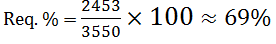QUESTION: 6

What will come in place of the question mark (?) in each of the following number series?

2, 8, 26, ?, 242

Solution: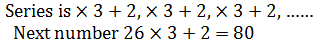QUESTION: 7

What will come in place of the question mark (?) in each of the following number series?

3, 4, 12, ?, 196

Solution: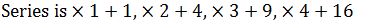QUESTION: 8

What will come in place of the question mark (?) in each of the following number series?

9, 17, ?, 65, 129

Solution: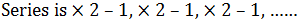QUESTION: 9

What will come in place of the question mark (?) in each of the following number series?

7, 13, ?, 35, 54

Solution: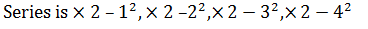QUESTION: 10

What will come in place of the question mark (?) in each of the following number series?

5, 3, 6, ?, 64.75

Solution: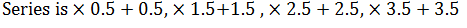QUESTION: 11

Study the following graph carefully to answer these questions.

Investments (in Rs. Lakh) of Two Business Partners A & B over the Years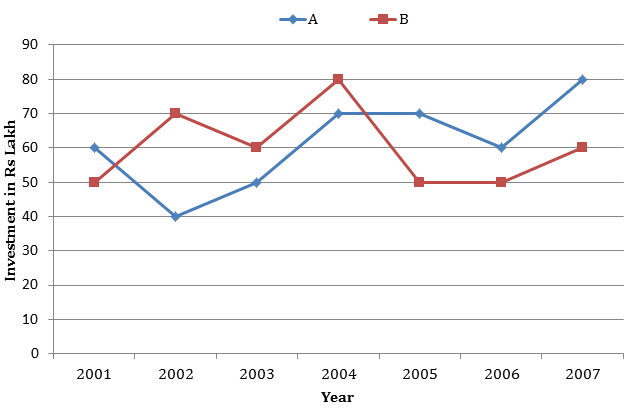Q. What was the per cent rise in A’s investment in 2004 from the previous year?

Solution: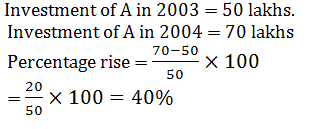QUESTION: 12

Study the following graph carefully to answer these questions.

Investments (in Rs. Lakh) of Two Business Partners A & B over the YearsQ. Investment of B in 2003 is approximately what per cent of his total investment for all the years together?

Solution: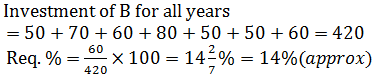QUESTION: 13

Study the following graph carefully to answer these questions.

Investments (in Rs. Lakh) of Two Business Partners A & B over the Years​Q. What is the ratio of total investment of A in 2001, 2002 and 2003 together to the total investment of B in these three years together respectively?

Solution: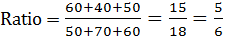QUESTION: 14

Study the following graph carefully to answer these questions.

Investments (in Rs. Lakh) of Two Business Partners A & B over the YearsQ. What was the per cent rise in investment of B in 2004 from 2001?

Solution: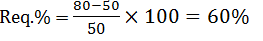QUESTION: 15

Study the following graph carefully to answer these questions.

Investments (in Rs. Lakh) of Two Business Partners A & B over the Years​Q. What was the per cent rise/fall in the total investment of A & B together from 2002 to 2005? (rounded off to two digits after decimal)

Solution:

Total investment of A and B in 2002 = 40 + 70 = 110
Total investment of A and B in 2005 = 50 + 70 = 120
Req.%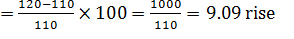QUESTION: 16

What will come in place of question mark (?) in the following questions?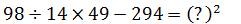Solution: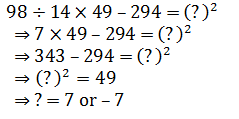QUESTION: 17

What will come in place of question mark (?) in the following questions?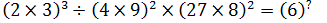Solution: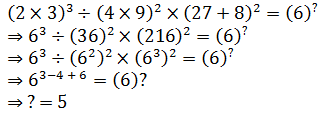QUESTION: 18

What will come in place of question mark (?) in the following questions?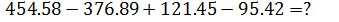Solution:

103.72

QUESTION: 19

What will come in place of question mark (?) in the following questions?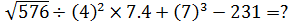Solution: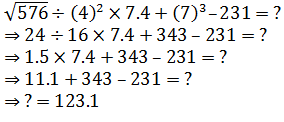QUESTION: 20

What will come in place of question mark (?) in the following questions?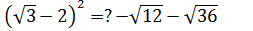Solution: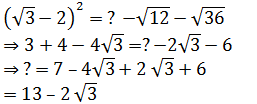QUESTION: 21

In the following questions two equations numbered I and II are given. You have to solve both the equations and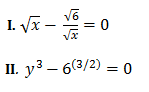Solution: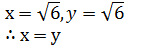QUESTION: 22

In the following questions two equations numbered I and II are given. You have to solve both the equations and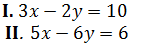Solution: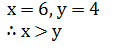QUESTION: 23

In the following questions two equations numbered I and II are given. You have to solve both the equations and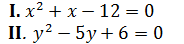Solution: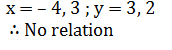QUESTION: 24

In the following questions two equations numbered I and II are given. You have to solve both the equations and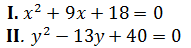Solution: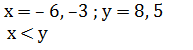QUESTION: 25

In the following questions two equations numbered I and II are given. You have to solve both the equations and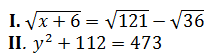Solution: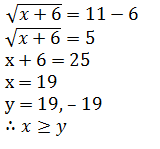QUESTION: 26

A merchant purchases a wrist watch for Rs. 450 and fixes its list price in such a way that after allowing a discount of 10%, he earns a profit of 20%. Then the list price of the watch is

Solution: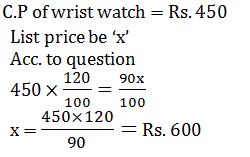QUESTION: 27

An article was sold at a profit of 12%. If the cost price would be 10% less and selling price would be Rs. 5.75 more, there would be profit of 30%. Then at what price it should be sold to make a profit of 20%?

Solution: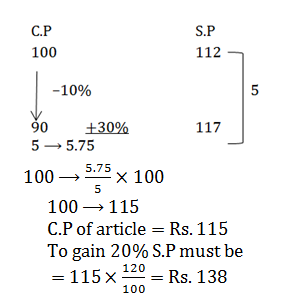QUESTION: 28

The simple interest on a sum of money is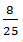of the sum. If the number of years is numerically half the rate percent per annum, then the rate percent per annum is

Solution: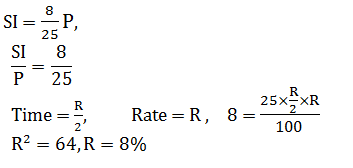QUESTION: 29

A sum of money is paid back in two annual installments of Rs. 17,640 each, allowing 5% compound interest compounded annually. The sum borrowed was

Solution: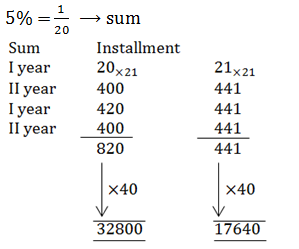QUESTION: 30

Three glasses of equal volume contain acid mixed with water. The ratio of acid and water are 2 : 3, 3 : 4 and 4 : 5 respectively. Contents of these glasses are poured in a large vessel. The ratio of acid and water in the large vessel is

Solution: